# Fdf

This project is about creating a simplified 3D graphic representation of a relief landscape or any other geometric images.
Alternatives To Fdf
Mvs Texturing768
2 months ago57otherC++
Algorithm to texture 3D reconstructions from multi-view stereo images
Probreg54616 months ago31January 08, 20225mitPython
Python package for point cloud registration using probabilistic model (Coherent Point Drift, GMMReg, SVR, GMMTree, FilterReg, Bayesian CPD)
Pianoplayer479
a year ago13January 24, 202111mitPython
Automatic fingering generator for piano scores
Pathfinding Visualizer Threejs364
3 months ago22Vue
A visualizer for pathfinding algorithms in 3D with maze generation, first-person view and device camera input.
3d Bin Container Packing29512 days ago27October 21, 202221apache-2.0Java
A variant of the Largest Area Fit First (LAFF) algorithm + brute force algorithm
Unity Teddy280
3 years ago1mitC#
Teddy algorithm implementation in Unity.
Connected Components 3d23018a month ago46June 24, 202213gpl-3.0C++
Connected components on discrete and continuous multilabel 3D & 2D images. Handles 26, 18, and 6 connected variants.
Delatin217
a year ago2iscJavaScript
A fast JavaScript terrain mesh generation tool based on Delaunay triangulation
3dlinedetection211
3 years ago4bsd-2-clauseC++
A simple and efficient 3D line detection algorithm for large scale unorganized point cloud
Pvgeo166
27 months ago68October 27, 201912bsd-3-clausePython
🌍 Python package of VTK-based algorithms to analyze geoscientific data and models
Alternatives To Fdf
Select To Compare

Alternative Project Comparisons

# FDF

[>man mlx]

[MAP_GENERATOR]

### Run on your local machine:

1. download `go_FDF` folder;
2. run `./fdf test_maps/map_name.fdf`;
3. follow the legend in the upper left corner of the screen.

## Some samples:

### Just 42: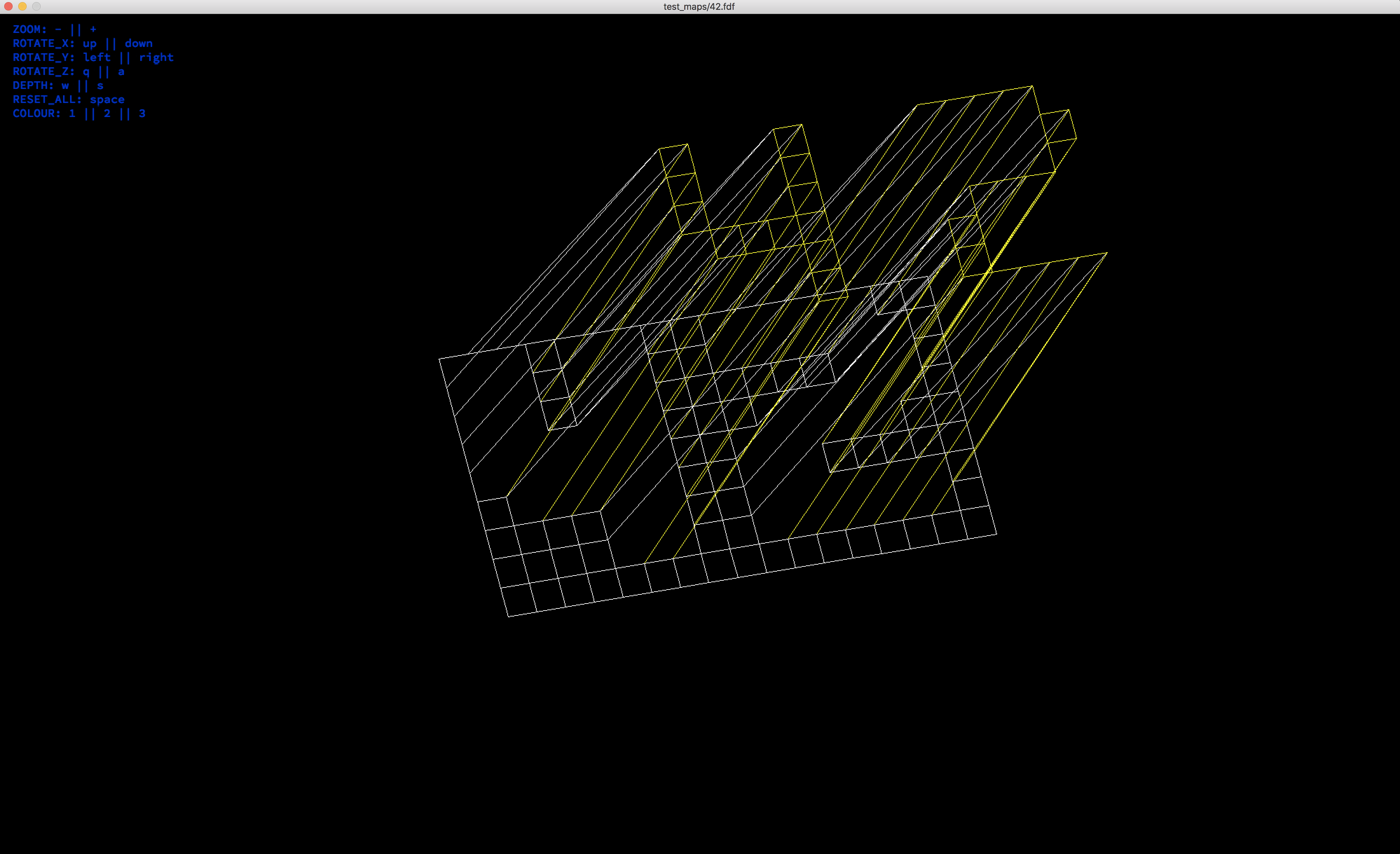### Mars landscape:### Pyramid: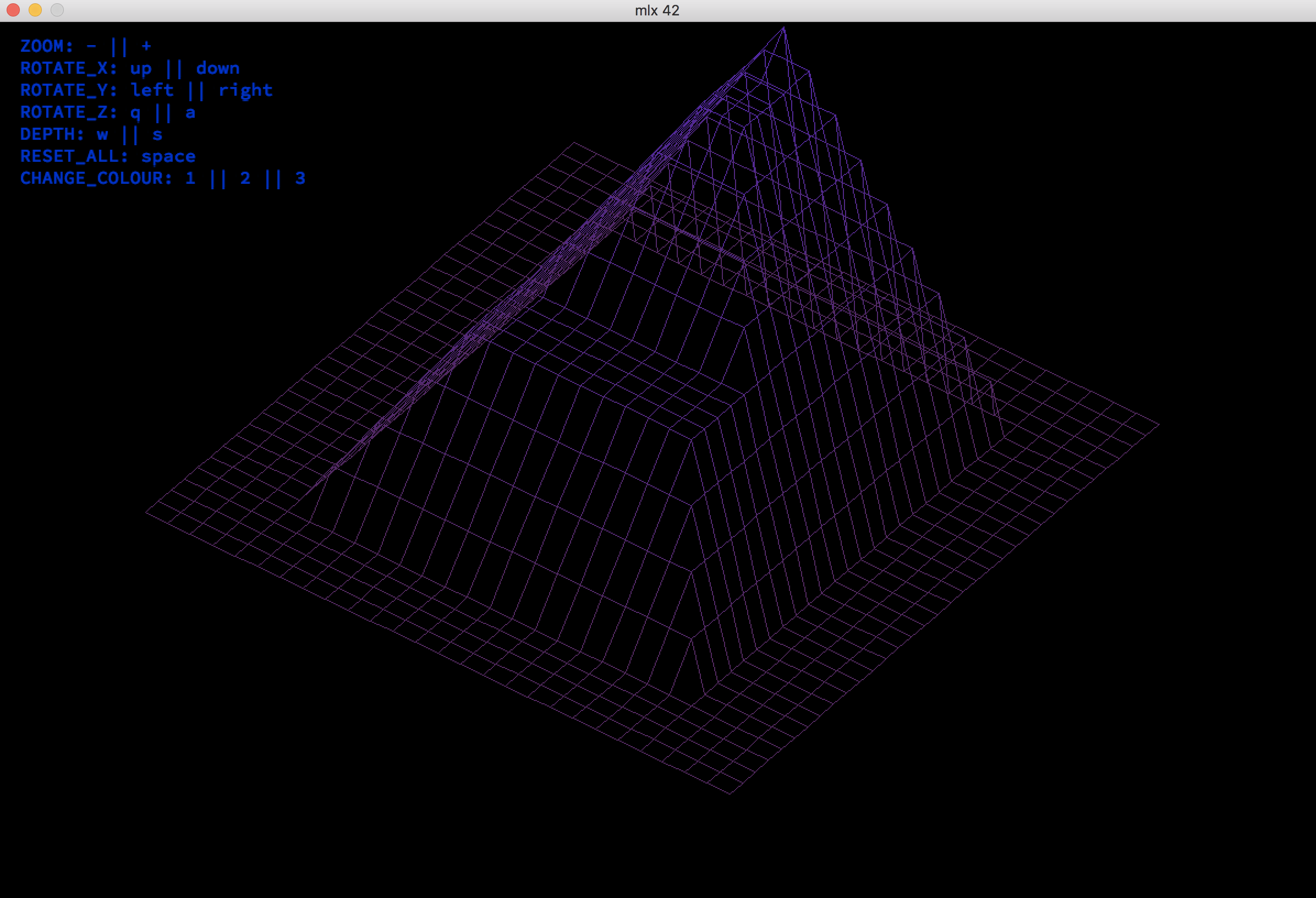### Mars landscape_2: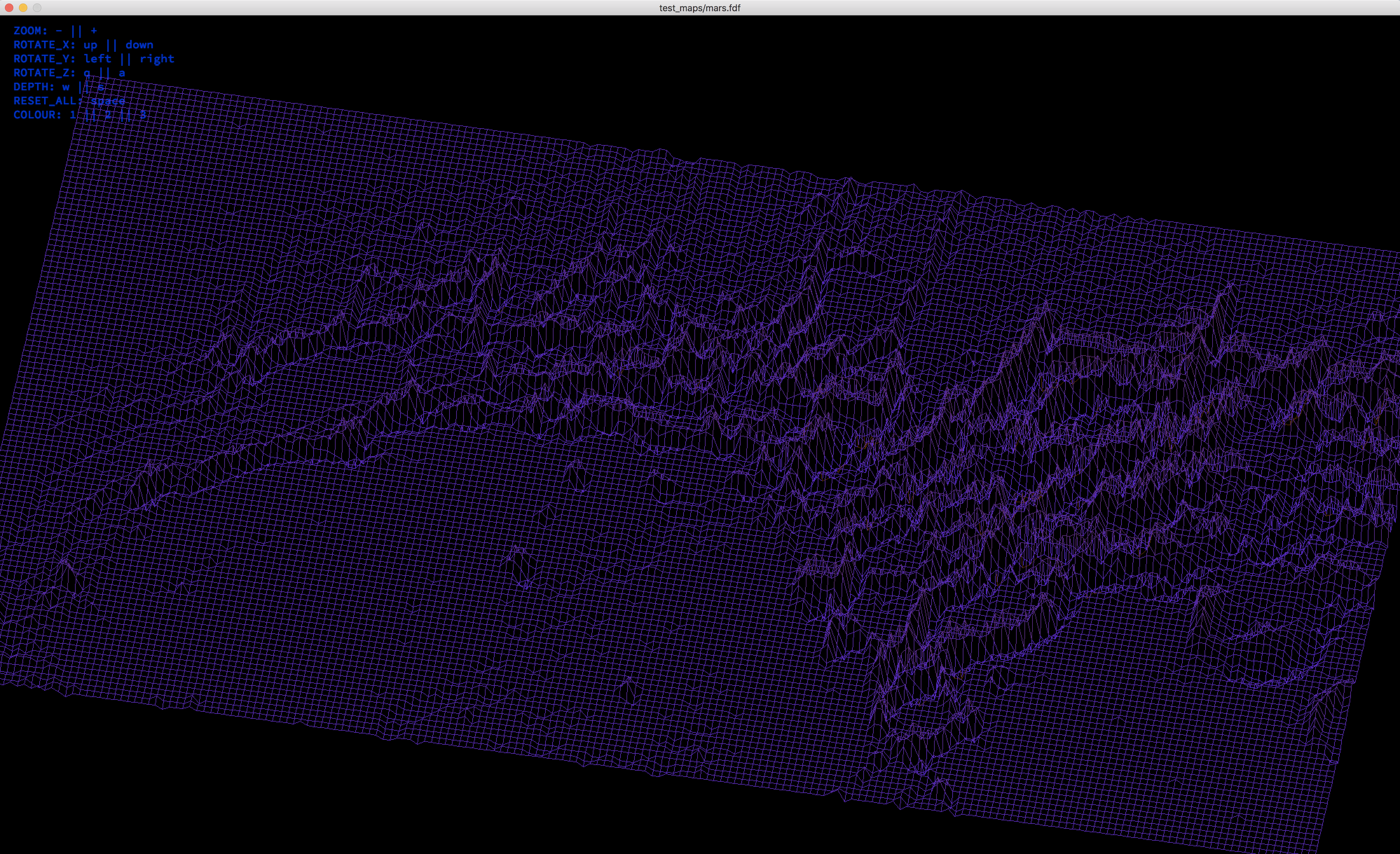### Pyra: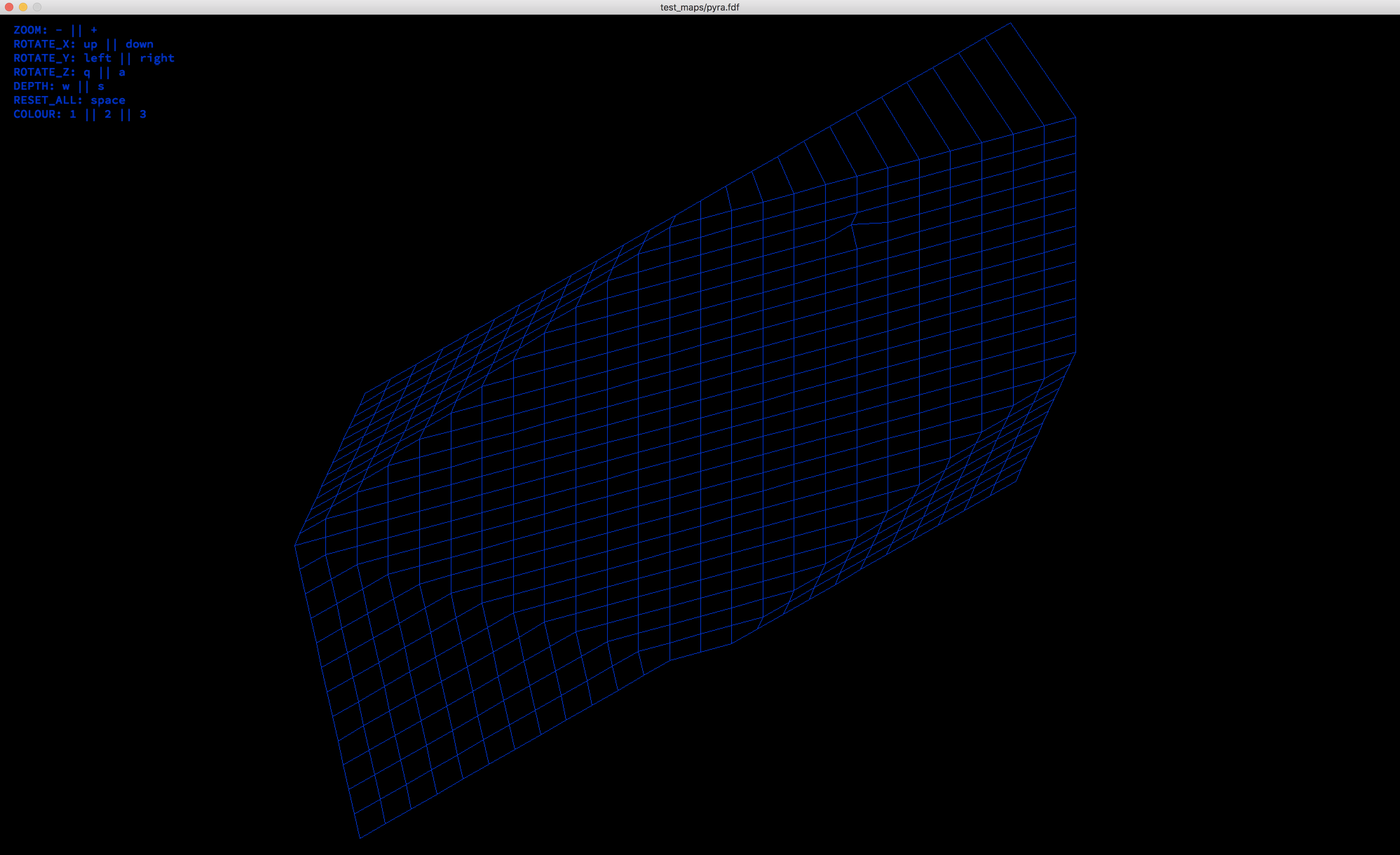### Ukraine (from NOAA (National Oceanic and Atmospheric Administration / USA)):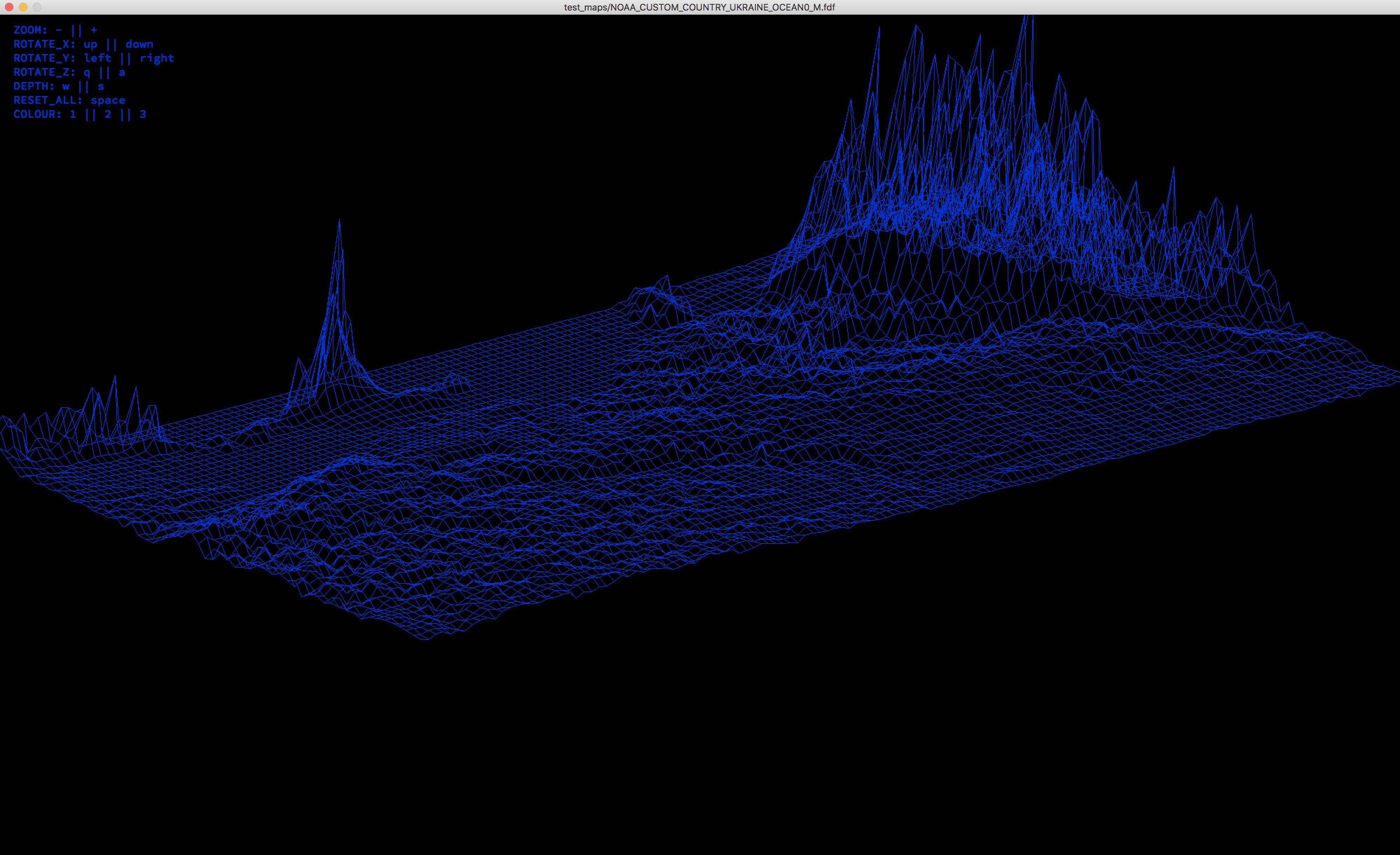### Ukraine (from NOAA (flatten ocean)):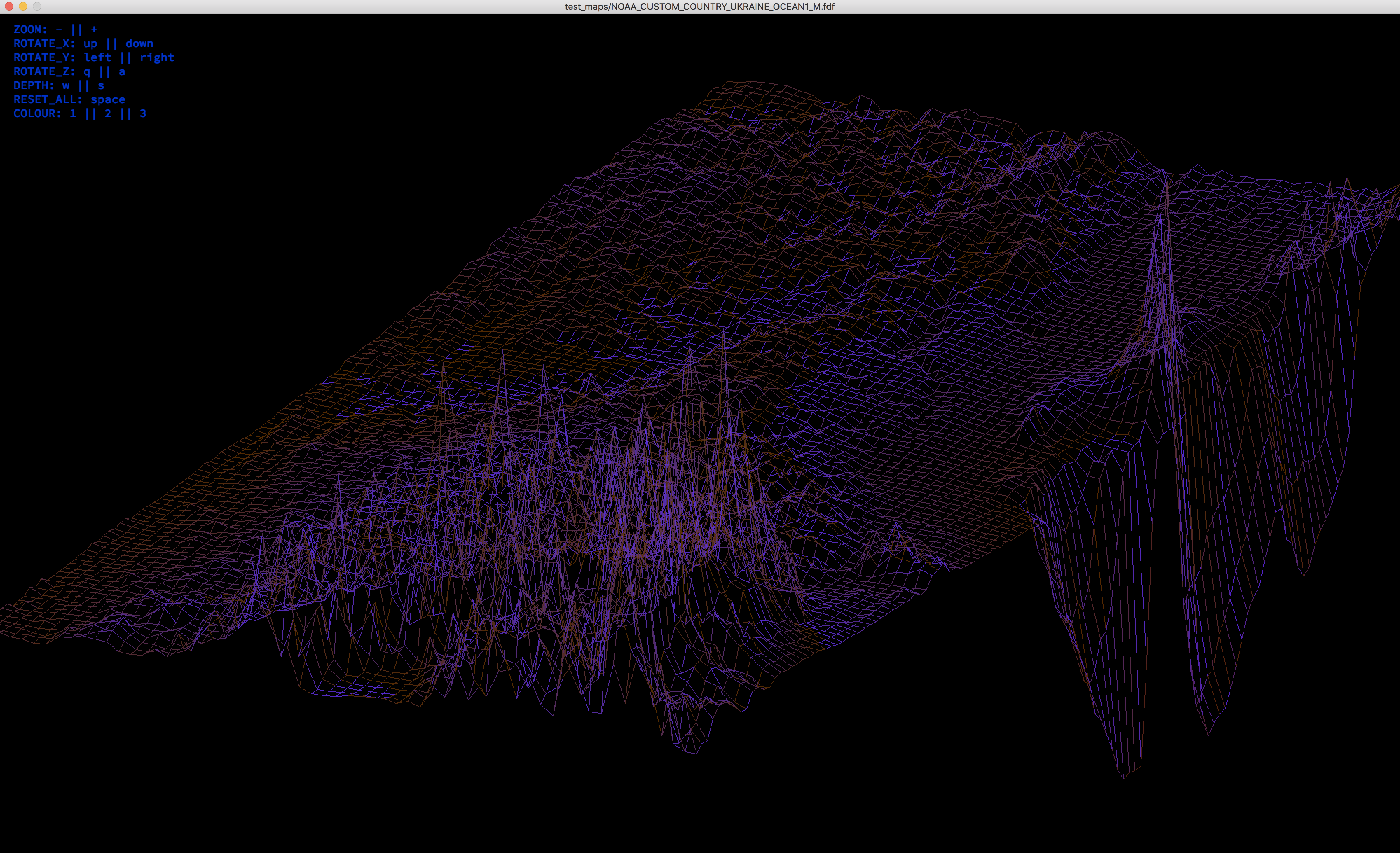The project implemented a pixel-by-pixel approach, so large cards work slowly. I concluded, that draw 1 pixel each time is misspend. In the following projects, i will change the approach. But for now to compile the project run `make`, u will get an executable file called `fdf` and then `./fdf test_maps/42.fdf`, where `42.fdf` is the map u want to visualize. Or you can download only the folder `go_FDF`, with executable file and maps, and simply run `./fdf test_maps/42.fdf`.

Files and folders needed for successful compilation:

``````.
├── fdf.c
├── get_next_line.c
├── error_manager.c
├── ft_atoi_base.c
├── ft_split.c
├── bresenham_line.c
├── transformations.c
├── actions.c
├── next_drawing.c
├── includes                        # .h files are here;
│   ├── get_next_line.h
│   └── fdf.h
├── libft                           # folder with my own library of some useful functions;
├── test_maps                       # some maps;
├── Makefile
└──
``````

The purpose of other folders:

``````├── LineUNIVERSE            # was created to improve my understanding of graphics in principle - a simple LINE and its 3d transformation in space;
├── TriangleUNIVERSE        # a simple TRIANGLE and its 3d transformation in space (i realized its rotation on 3 axes (x, y, z) in a loop);
├── go_FDF                  # contains executable called `fdf` and maps;
├── go_MinilibX             # here I tested the graphics library and drew some lines;
├── minilibx                # the sources of the graphic library;
├── minilibx_macos          # the sources of the graphic library_macos;
├── screenshots             # some additional examples of how program does work;
└──
``````

Do not forget that you can use the keyboard for various actions on the rendered image (see the legend in the graphics window). Realized such functions as: zooming; rotation around the axis X, axis Y and axis Z; changing the height of the figure; changing the color; resetting to the original rendering.

The key algorithm of this project is Bresenham's line algorithm. Below you can find my analysis of this algorithm.

## Bresenham's line algorithm

A line connects two points. It is a basic element in graphics. To draw a line, you need two points between which you can draw a line. And an algorithm to do this. Bresenham's line algorithm can use integer calculations, so it works faster than approaches with floats. This algorithm is needed in order to draw a line at any angle and in any direction (and not just proceeding from the origin). On our screen the origin is in the upper left corner of the screen: `y` increases, when we step down, `x` grows as we move to the right. Going through understanding the algorithm, becomes clear, that we have two cases: 1) `deltaX(x1 - x0) > deltaY(y1 - y0)` : `x` is increased each iteration, about `y` we should make decision - keep it same or increase by step (1 or -1: depends on direction of the line). This case draws lines only if the inclination of the line is less then 45 degrees. 2) `deltaX(x1 - x0) < deltaY(y1 - y0)` : `y` is increased each iteration, about `x` we should make decision - keep it same or increase by step (1 or -1: depends on direction of the line). This case draws lines only if the inclination of the line is more then 45 degrees. Let's visualize it.

### Case 1. deltaX(x1 - x0) > deltaY(y1 - y0) (x++)

Here is the structure, that i use (for your understanding of the variable names):

``````# define WIDTH 1000
# define HEIGHT 600

typedef struct			s_env
{
void			*mlx;
void			*win;
int			step_x;
int			step_y;
int			dx;
int			dy;
int			x0;
int			x1;
int			y0;
int			y1;
int			line_color;
}				t_env;
``````

And the implementation of Bresenham's line algorithm (i split the code into several functions for clarity):

``````/* if dy <= dx */
static	void		ft_draw_dx(t_env *e)
{
int	error;
int	i;
int	x;
int	y;

error = (e->dy << 1) - e->dx;
x = e->x0 + e->step_x;
y = e->y0;
i = 1;
mlx_pixel_put(e->mlx, e->win, e->x0, e->y0, e->line_color);
while (i <= e->dx)
{
if (error > 0)
{
y += e->step_y;
error += (e->dy - e->dx) << 1;
}
else
error += e->dy << 1;
mlx_pixel_put(e->mlx, e->win, x, y, e->line_color);
x += e->step_x;
i++;
}
}

/* if dy >= dx */
static	void		ft_draw_dy(t_env *e)
{
int	error;
int	i;
int	x;
int	y;

error = (e->dx << 1) - e->dy;
y = e->y0 + e->step_y;
x = e->x0;
i = 1;
mlx_pixel_put(e->mlx, e->win, e->x0, e->y0, e->line_color);
while (i <= e->dy)
{
if (error > 0)
{
error += (e->dx - e->dy) << 1;
x += e->step_x;
}
else
error += e->dx << 1;
mlx_pixel_put(e->mlx, e->win, x, y, e->line_color);
y += e->step_y;
i++;
}
}

/* making a decision: deltaX > deltaY || deltaX > deltaY */
/* also we should figure out the value of step (1 or -1) */
void				bresenham_line(t_env *e)
{
e->dx = abs(e->x1 - e->x0); /* delta x */
e->dy = abs(e->y1 - e->y0); /* delta y */
e->step_x = e->x1 >= e->x0 ? 1 : -1; /* stepx if line (from left to right) = +1 ; else = -1 */
e->step_y = e->y1 >= e->y0 ? 1 : -1; /* stepy if line (from up to down) = +1 ; else = -1 */
if (e->dx > e->dy)
ft_draw_dx(e);
else
ft_draw_dy(e);
}
``````

Simple main.c (just to see how the algorithm works when dy <= dx):

``````int			main(void)
{
int 	color;
t_env 	*e;

if (!(e = malloc(sizeof(t_env))))
return (0);
color = 8388352;
e->mlx = mlx_init();
e->win = mlx_new_window(e->mlx, WIDTH, HEIGHT, "mlx 42");
e->x0 = 0;
e->x1 = WIDTH;
e->y0 = 0;
e->y1 = HEIGHT;
e->line_color = 8388352;
bresenham_line(e);
mlx_loop(e->mlx);
free(e);
return (0);
}
``````

I am running:

``````gcc -I /Users/kprytkov/FDF/go_MinilibX main.c -L /usr/local/lib -lmlx -framework OpenGL -framework Appkit
``````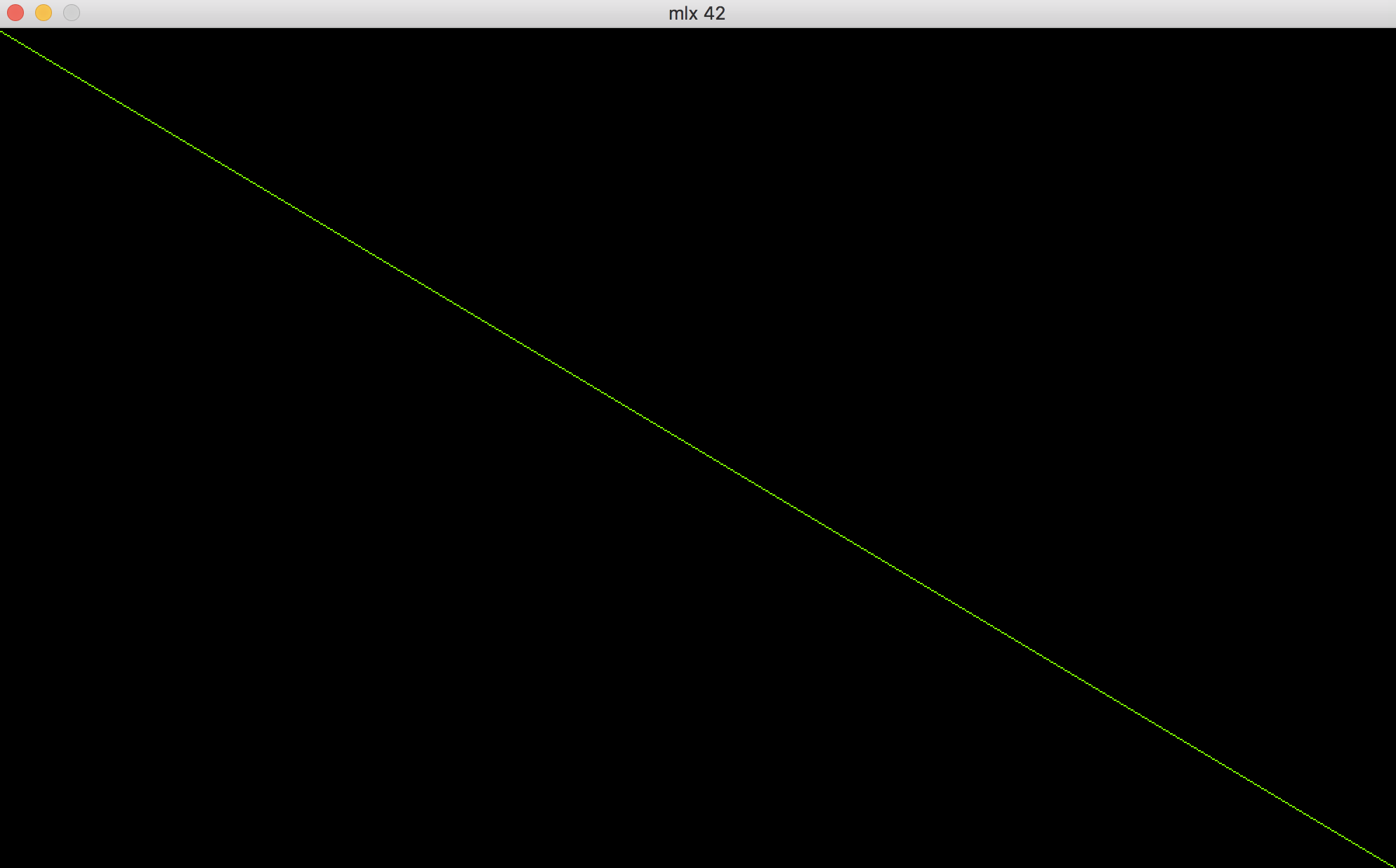Modify your main.c in this way and you will see the entire spectrum of the action of the function `ft_draw_dx()`:

``````int			main(void)
{
int 	color;
t_env 	*e;

if (!(e = malloc(sizeof(t_env))))
return (0);
color = 8388352;
e->mlx = mlx_init();
e->win = mlx_new_window(e->mlx, WIDTH, HEIGHT, "mlx 42");
for (int j = 0; j < HEIGHT; j++)
{
e->x0 = 0 + WIDTH / 2;
e->x1 = WIDTH;
e->y0 = 0 + HEIGHT / 2;
e->y1 = j;
e->line_color = j;
bresenham_line(e);
}
for (int j = 0; j < HEIGHT; j++)
{
e->x0 = WIDTH / 2;
e->x1 = 0;
e->y0 = HEIGHT / 2;
e->y1 = j;
e->line_color = j;
bresenham_line(e);
}
mlx_loop(e->mlx);
free(e);
return (0);
}
``````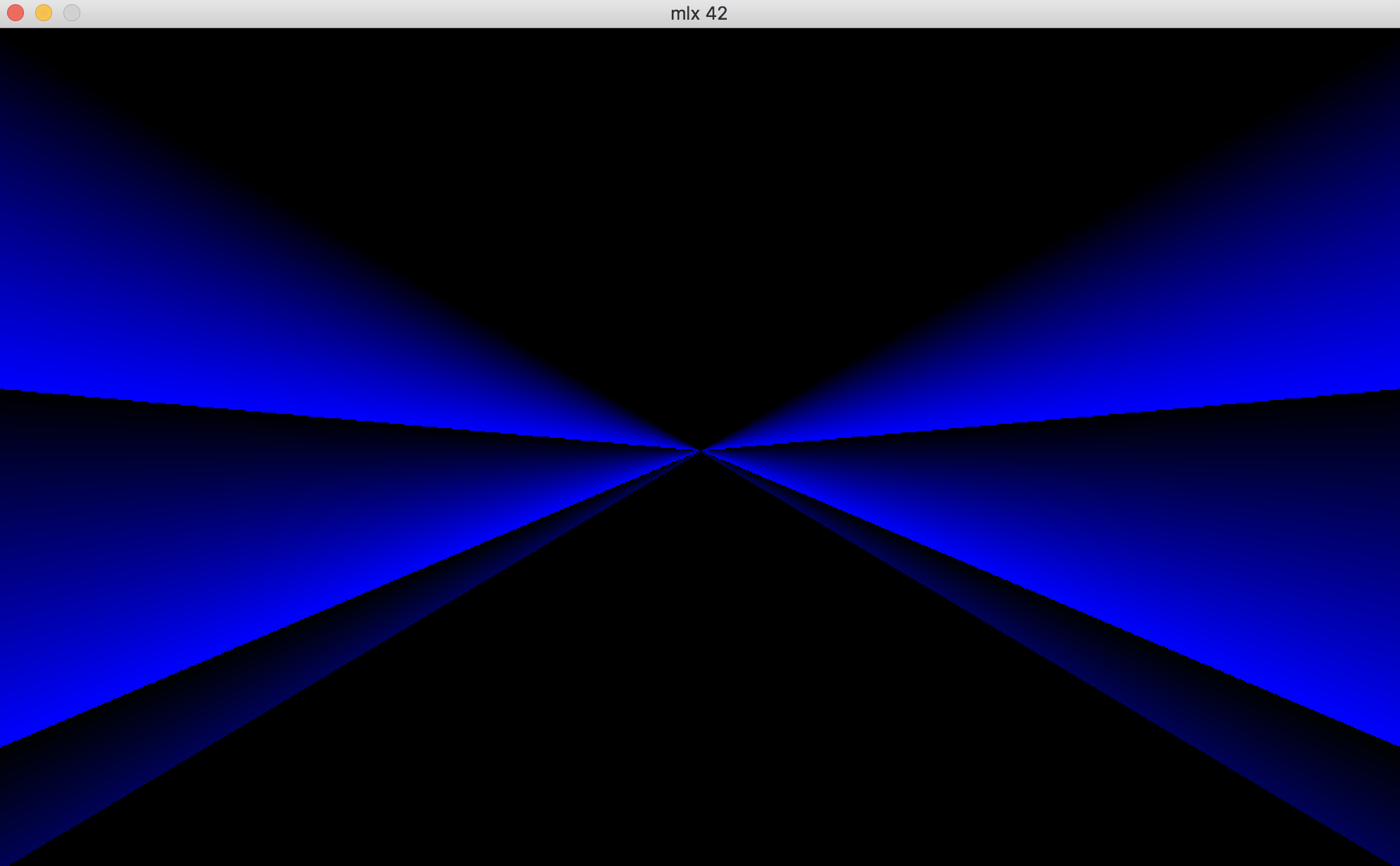### Case 2. deltaX(x1 - x0) < deltaY(y1 - y0) (y++)

See how ft_draw_dy() is working (fn random() does make decisions about line's color):

``````int			main(void)
{
int 	color;
t_env 	*e;

if (!(e = malloc(sizeof(t_env))))
return (0);
color = 8388352;
e->mlx = mlx_init();
e->win = mlx_new_window(e->mlx, WIDTH, HEIGHT, "mlx 42");
for(int j = 0; j < WIDTH; j++)
{
e->x0 = WIDTH / 2;
e->x1 = j;
e->y0 = HEIGHT / 2;
e->y1 = HEIGHT;
e->line_color = random();
bresenham_line(e);
}
for(int j = 0; j < WIDTH; j++)
{
e->x0 = WIDTH / 2;
e->x1 = j;
e->y0 = HEIGHT / 2;
e->y1 = 0;
e->line_color = random();
bresenham_line(e);
}
mlx_loop(e->mlx);
free(e);
return (0);
}
``````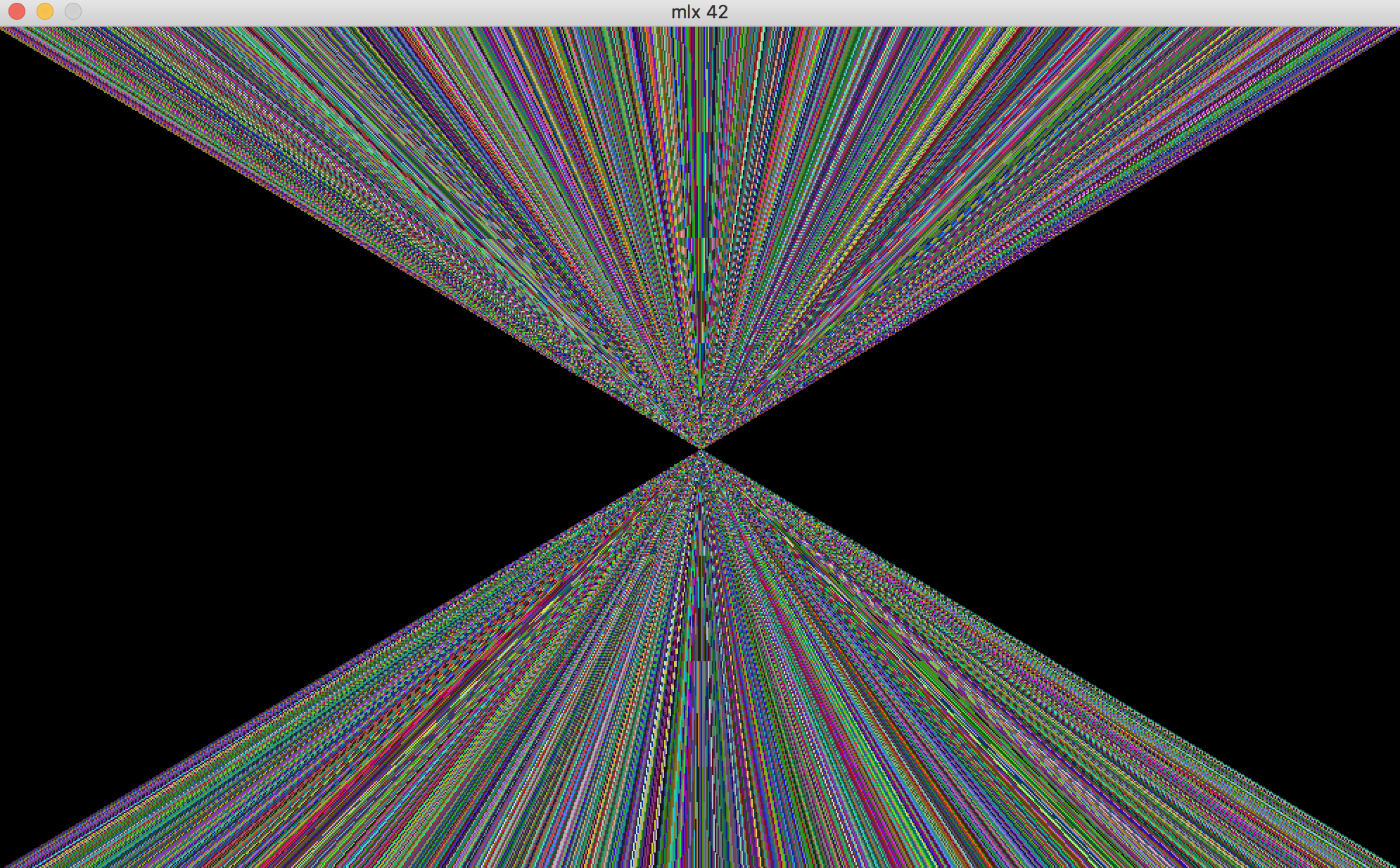And just for fun:

``````int			main(void)
{
int 	color;
t_env 	*e;

if (!(e = malloc(sizeof(t_env))))
return (0);
color = 8388352;
e->mlx = mlx_init();
e->win = mlx_new_window(e->mlx, WIDTH, HEIGHT, "mlx 42");
for (int i = 0; i <= 500; i++)
{
e->x0 = random() % WIDTH - 1;
e->x1 = random() % WIDTH - 1;
e->y0 = random() % HEIGHT - 1;
e->y1 = random() % HEIGHT - 1;
e->line_color = random();
bresenham_line(e);
}
mlx_loop(e->mlx);
free(e);
return (0);
}
``````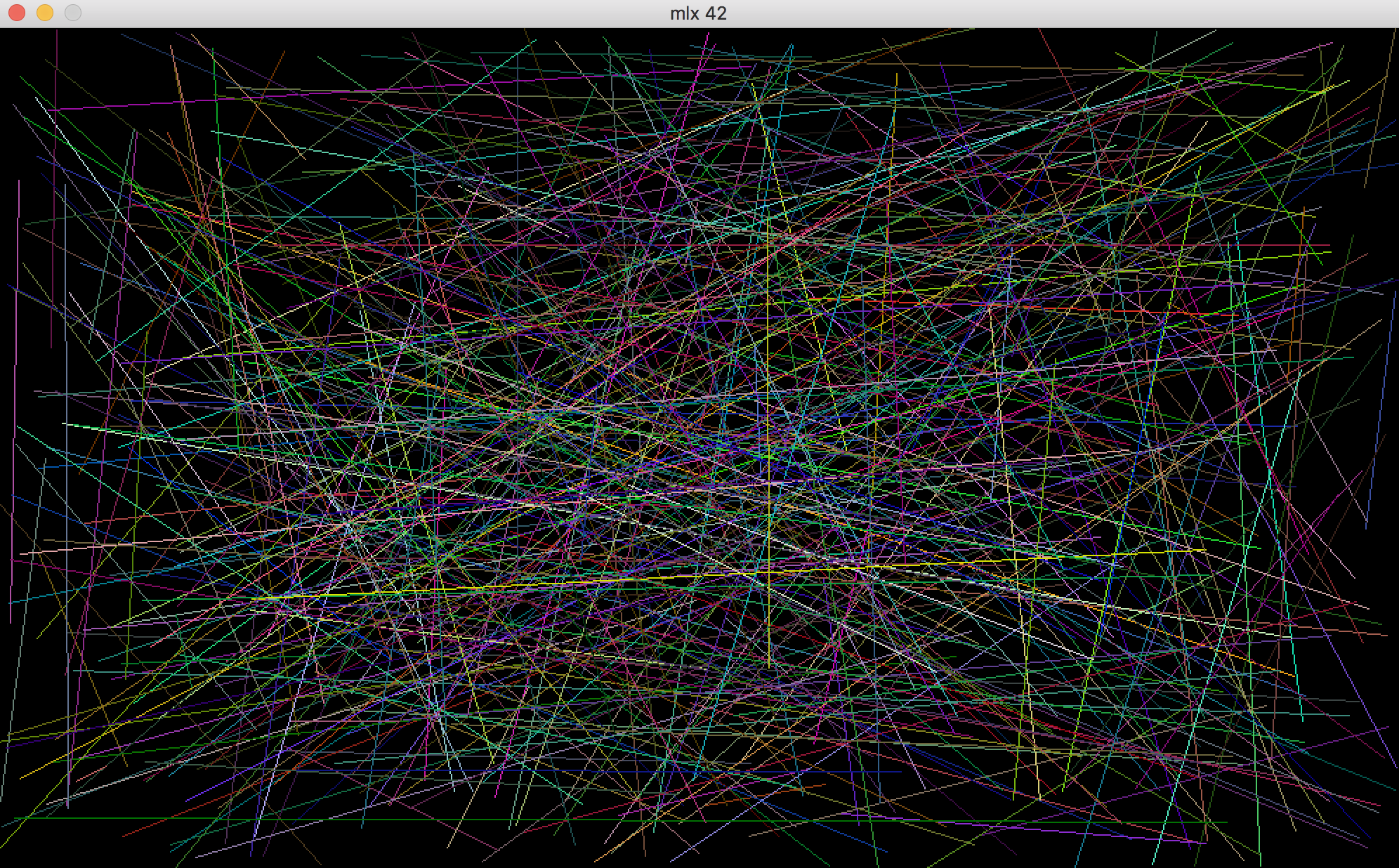view code here: FDF/go_MinilibX/